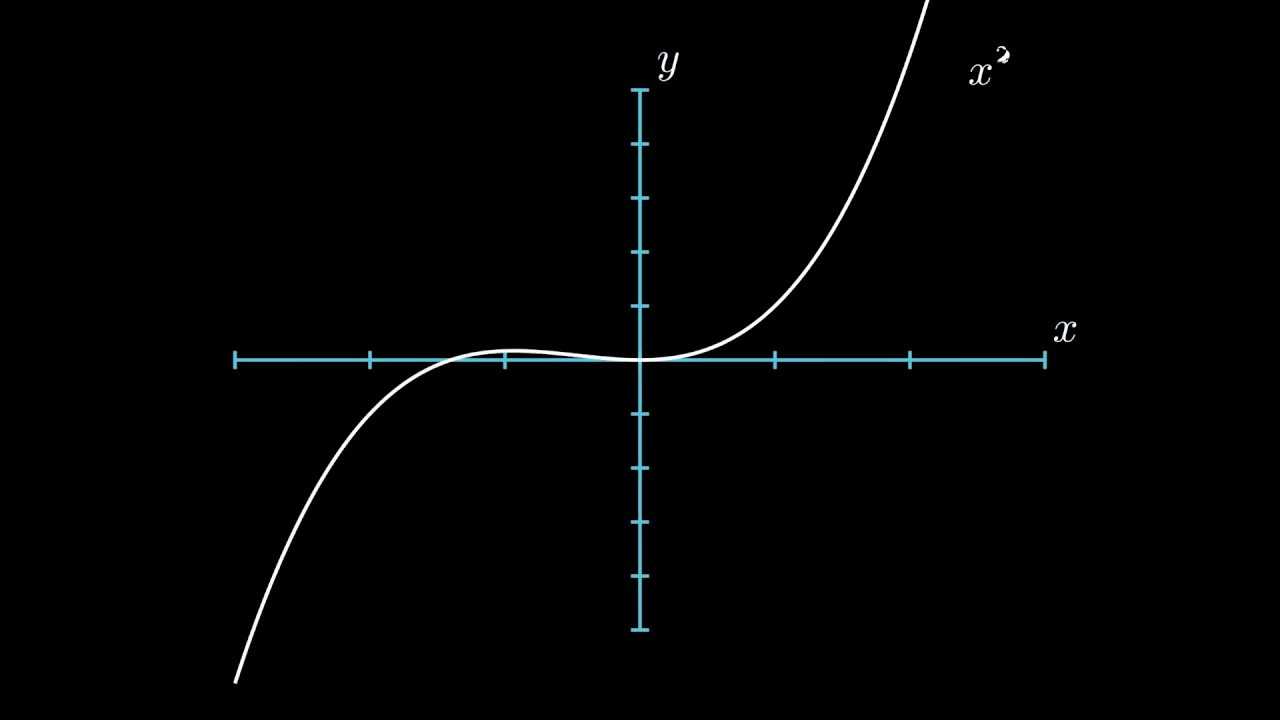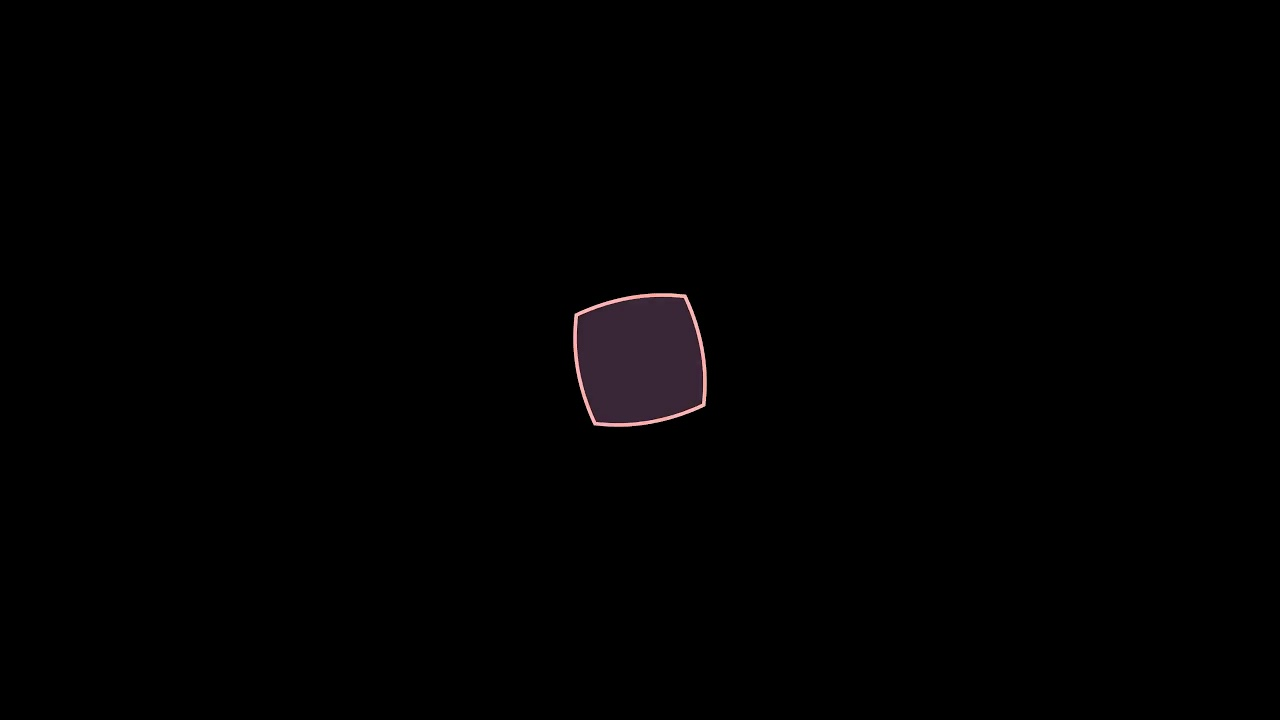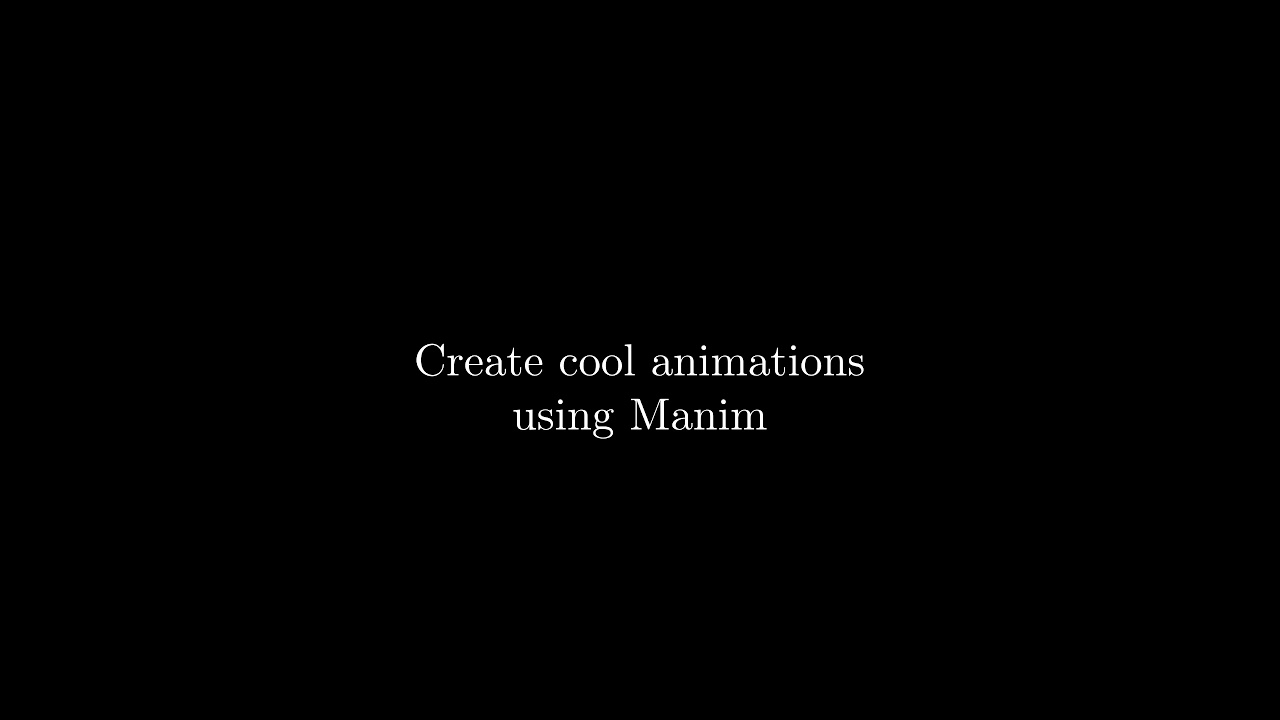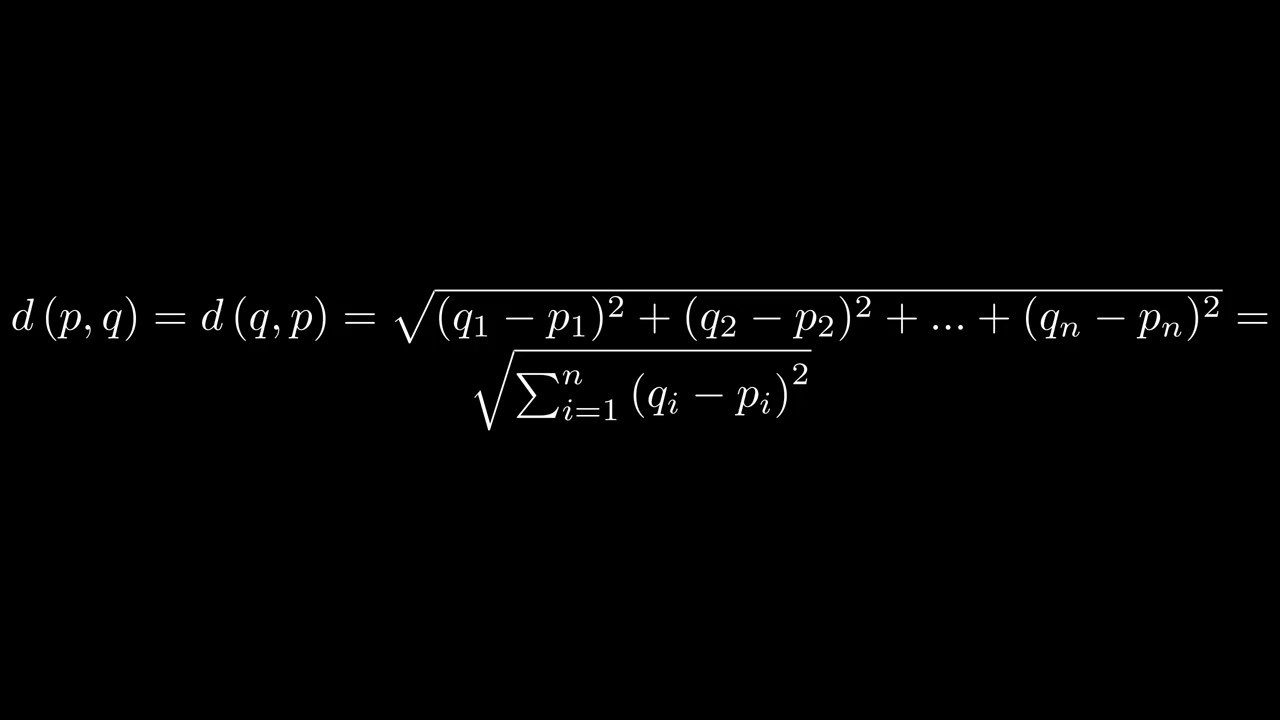# Manim Examples

Examples on how to use Manim
Alternatives To Manim Examples
Aachartkit Swift2,304
12 months ago17May 31, 2022143mitSwift
📈📊📱💻🖥️An elegant modern declarative data visualization chart framework for iOS, iPadOS and macOS. Extremely powerful, supports line, spline, area, areaspline, column, bar, pie, scatter, angular gauges, arearange, areasplinerange, columnrange, bubble, box plot, error bars, funnel, waterfall and polar chart types. 极其精美而又强大的现代化声明式数据可视化图表框架,支持柱状图、条形图、折线图、曲线图、折线填充图、曲线填充图、气泡图、扇形图、环形图、散点图、雷达图、混合图等各种类型的多达几十种的信息图图表,完全满足工作所需.
Vizzu Lib1,80824 days ago28November 22, 202311apache-2.0JavaScript
Library for animated data visualizations and data stories.
React Native Graph1,54223 months ago4June 07, 202326mitTypeScript
📈 Beautiful, high-performance Graphs and Charts for React Native
Easinginterpolator1,080
2 years agoJava
Thirty-one different easing animation interpolators for Android.
Resonance1,018455 years ago31February 17, 2019mitJavaScript
:black_medium_small_square:Resonance | 5kb React animation library
Ipyvizzu87532 months ago23October 12, 202314apache-2.0Python
Build animated charts in Jupyter Notebook and similar environments with a simple Python syntax.
Hdrpvatexample501
VAT (Vertex Animation Texture) with Unity Shader Graph and Visual Effect Graph
Graphvizanim477112 months ago7April 03, 20194gpl-3.0Python
A tool to create animated graph visualizations, based on graphviz.
Rthreejs277136a year ago4January 21, 202036otherJavaScript
Three.js widgets for R and shiny
Possumwood206
2 years ago2mitC++
Possumwood is a graph-based procedural authoring tool, in concept not dissimilar to popular CG packages like Houdini, Blender or Maya. It is intended to serve as a sandbox for computer graphics algorithms and libraries, providing a user-friendly and coding-free UI for libraries that would otherwise be inaccessible for an average user.
Alternatives To Manim Examples
Select To Compare

Alternative Project Comparisons

# Manim Examples## Installation

For installing Manim on your system I recommend following the Installation guide from the Manim documentation.

Now that you have installed everything correctly you're ready to write your first application.

from manim import *

class SquareToCircle(Scene):
def construct(self):
# Creating shapes
circle = Circle()
square = Square()

#Showing shapes
self.play(Create(square))
self.play(Transform(square, circle))

manim square_to_circle.py SquareToCircle -p -qlLets break this down step-by-step:

• The import statement on top imports everything needed to use Manim.
• For running animations, you have to create a class that inherits from Manims Scene class.
• Inside the class you need to create the construct method. The construct method is essentially the main method for the class. In it you can write all the code for the animation.
• Inside the construct method I first created two MObjects (Manim Objects) – a circle and a square. Lastly I displayed the shapes using the play method.

We can also modify the appearance of the objects by adding a few lines of code:

from manim import *

class SquareToCircleWithModifications(Scene):
def construct(self):
circle = Circle()
square = Square()
square.flip(RIGHT)
square.rotate(-3 * TAU / 8)
circle.set_fill(PINK, opacity=0.5)

self.play(Create(square))
self.play(Transform(square, circle))## Displaying text

Displaying text is also pretty straight forward.

from manim import *

class displayText(Scene):
def construct(self):
# Create Text objects
first_line = Text('Create cool animations')
second_line = Text('using Manim')
third_line = Text('Try it out yourself.', color=RED)

# Position second line underneath first line
second_line.next_to(first_line, DOWN)

# Displaying text
self.wait(1)
self.play(Write(first_line), Write(second_line))
self.wait(1)
self.wait(2)


In order to display text you need to create a Text object and pass it the text you want to write to the screen. After that you can display the text using the play method and some animation.## Math equations

Math equations can be written in Manim using LaTeX – a typesetting system widely used in academia. On of LaTeX big advantages is that it allows you to create good looking math equation with an intuitive system.

I won't go over LaTeX in this article but if you are curious there are lots of great tutorials out there.

For equations instead of using a Text object you need to use a Tex object. When making an equation you need to put a $at the start and end of the text. text = Text('some text') equation = Tex('$some equation$')  You can add symbols like the summation or integral symbols with two backslashes. alpha = Tex('$\\alpha$')  Displaying some text and an equation could look like the following: from manim import * class displayEquations(Scene): def construct(self): # Create Tex objects first_line = Text('Manim also allows you') second_line = Text('to show beautiful math equations') equation = Tex('$d\\left(p, q\\right)=d\\left(q, p\\right)=\\sqrt{(q_1-p_1)^2+(q_2-p_2)^2+...+(q_n-p_n)^2}=\\sqrt{\\sum_{i=1}^n\\left(q_i-p_i\\right)^2}\$')

# Position second line underneath first line
second_line.next_to(first_line, DOWN)

# Displaying text and equation
self.play(Write(first_line), Write(second_line))
self.wait(1)
self.wait(3)## Creating graphs

Manim also allows us to create and display graphs.

from manim import *

class CreateGraph(Scene):
def construct(self):
axes = Axes(
x_range=[-3, 3],
y_range=[-5, 5],
axis_config={"color": BLUE},
)

# Create Graph
graph = axes.get_graph(lambda x: x**2, color=WHITE)
graph_label = axes.get_graph_label(graph, label='x^{2}')

graph2 = axes.get_graph(lambda x: x**3, color=WHITE)
graph_label2 = axes.get_graph_label(graph2, label='x^{3}')

# Display graph
self.play(Create(axes), Create(graph), Write(graph_label))
self.wait(1)
self.play(Transform(graph, graph2), Transform(graph_label, graph_label2))
self.wait(1)As you can see to create a graph you need to create a method that returns a y value for every x value it gets. In the code above I used lambda functions to specify them but you can also use any other method. After you have created the method you need to pass it to axes.get_graph, which creates a mobject out of the method.

Note that the method only specifies how the graph should look like and doesn't actually calculate any values yet.

## Author

Gilbert Tanner

Popular Graph Projects
Popular Animation Projects
Popular Computer Science Categories
Related Searches

Get A Weekly Email With Trending Projects For These Categories
No Spam. Unsubscribe easily at any time.
Python
Graph
Animation
Math
Latex
Scene